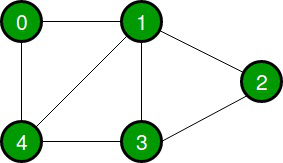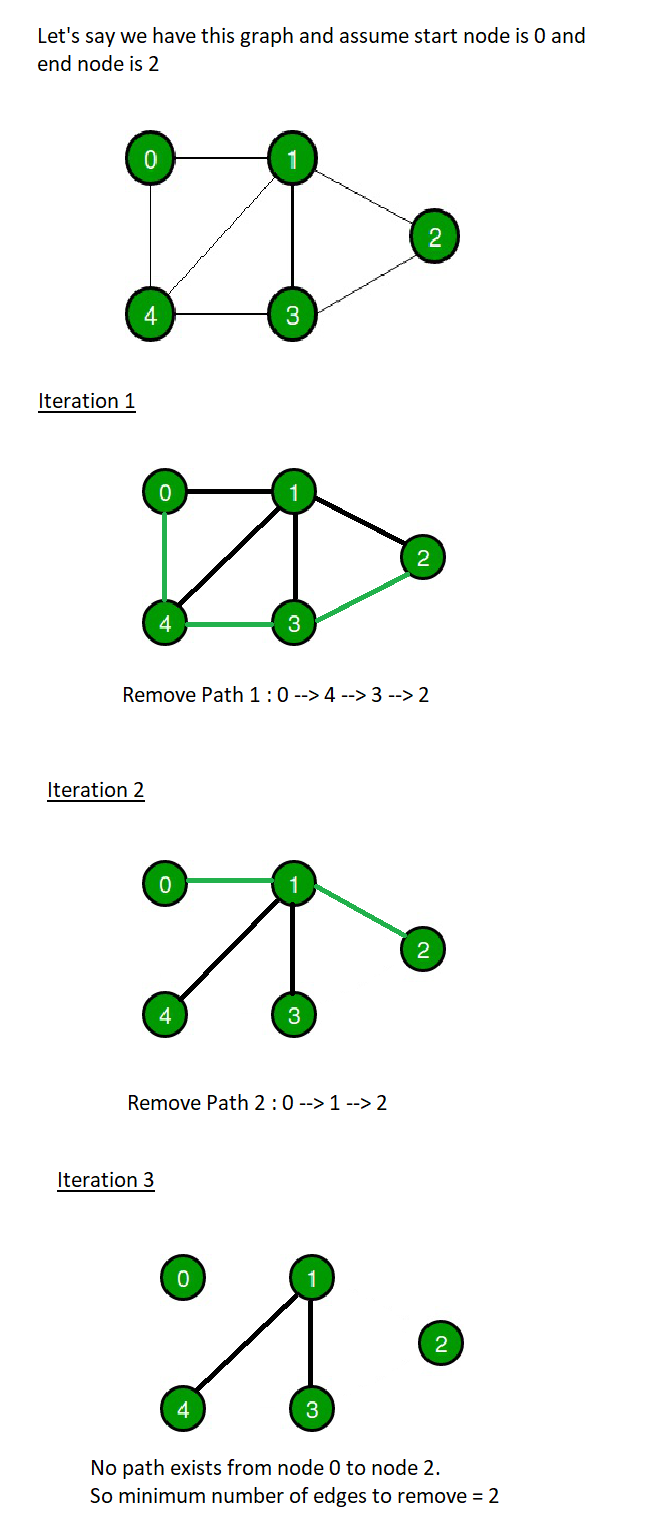# Java Program to Find Minimum Number of Edges to Cut to Make the Graph Disconnected

• Last Updated : 24 Jun, 2021

Given a connected graph, the task is to find the minimum number of edges to cut/remove to make the given graph disconnected.

A graph is disconnected if there exists at least two vertices of the graph that are not connected by a path.

Attention reader! Don’t stop learning now. Get hold of all the important DSA concepts with the DSA Self Paced Course at a student-friendly price and become industry ready.  To complete your preparation from learning a language to DS Algo and many more,  please refer Complete Interview Preparation Course.

In case you wish to attend live classes with experts, please refer DSA Live Classes for Working Professionals and Competitive Programming Live for Students.

Examples:

Input:Output: Minimum Number of Edges to Remove = 2

Approach:

The approach to this problem is to find the number of paths in the edge disjoint set of paths from a start vertex to an end vertex in the graph. Edge-disjoint set of paths is a set of paths having no common edge between any two paths.

1. Pick one node as start node and another node as end node.
2. Start BFS from the start node and check if a path exists from start node to end node.
3. If yes, then remove all the edges from that path and run BFS again.
4. Repeat step 2 and 3 until there’s no path exists from start node to end node.
5. Return the number of times a path is deleted.

Explanation with example:Code:

## Java

 `// Java Program to Find``// Minimum Number of Edges``// to Cut to make the``// Graph Disconnected` `import` `java.util.*;` `public` `class` `GFG {` `    ``// Function to find the min number of edges``    ``public` `static` `int` `minEdgesRemoval(``int``[][] edges, ``int` `n)``    ``{``        ``// Initialize adjacency list for Graph``        ``Map > graph``            ``= ``new` `HashMap >();` `        ``// Initializing starting and ending vertex``        ``int` `start = edges[``0``][``0``];``        ``int` `end = edges[``0``][``1``];` `        ``// Create adjacency list of the graph``        ``for` `(``int` `i = ``0``; i < n; i++) {``            ``int` `n1 = edges[i][``0``];``            ``int` `n2 = edges[i][``1``];``            ``List li;` `            ``// Add edges node 1``            ``if` `(graph.containsKey(n1)) {``                ``li = graph.get(n1);``            ``}``            ``else` `{``                ``li = ``new` `ArrayList();``            ``}``          ` `            ``li.add(n2);``            ``graph.put(n1, li);` `            ``// Add edges node 2``            ``if` `(graph.containsKey(n2)) {``                ``li = graph.get(n2);``            ``}``            ``else` `{``                ``li = ``new` `ArrayList();``            ``}``          ` `            ``li.add(n1);``            ``graph.put(n2, li);``        ``}` `        ``// Variable to count the number of paths getting``        ``// deleted``        ``int` `deleteEdgeCount = ``0``;``      ` `        ``while` `(``true``) {` `            ``// bfsTraversalPath is the BFS path from start``            ``// to end node It is a map of parent vertex and``            ``// child vertex``            ``Map bfsTraversalPath = bfs(graph, start);` `            ``// If end is present on the path from start node``            ``// then delete that path and increment``            ``// deleteEdgeCount``            ``if` `(bfsTraversalPath.containsKey(end)) {``              ` `                ``deleteEdgeCount++;``                ``int` `parent = bfsTraversalPath.get(end);``                ``int` `currEnd = end;``              ` `                ``// Delete all the edges in the current path``                ``while` `(parent != -``1``)``                ``{``                    ``deleteEdge(graph, parent, currEnd);``                    ``deleteEdge(graph, currEnd, parent);``                    ``currEnd = parent;``                    ``parent = bfsTraversalPath.get(currEnd);``                ``}``            ``}` `            ``// If end is not present in the path``            ``// then we have a disconnected graph.``            ``else` `{``                ``break``;``            ``}``        ``}` `        ``return` `deleteEdgeCount;``    ``}` `    ``// Function to delete/remove an edge``    ``private` `static` `void` `deleteEdge(Map > graph,``               ``Integer start, Integer end)``    ``{` `        ``List list = graph.get(start);``        ``list.remove(end);``    ``}` `    ``// Function for BFS Path``    ``private` `static` `Map``    ``bfs(Map > graph, ``int` `start)``    ``{` `        ``// Map for BFS Path``        ``Map bfsTraversalPath``            ``= ``new` `HashMap();` `        ``// Array for marking visited vertex``        ``List visited = ``new` `ArrayList();` `        ``// Array for BFS``        ``List queue = ``new` `ArrayList();``      ` `        ``int` `qStartIndex = ``0``;``      ` `        ``bfsTraversalPath.put(start, -``1``);``        ``queue.add(start);``      ` `        ``while` `(qStartIndex < queue.size())``        ``{``            ``int` `curr = queue.get(qStartIndex++);``            ``visited.add(curr);``          ` `            ``for` `(``int` `k : graph.get(curr))``            ``{``                ``if` `(!visited.contains(k))``                ``{``                    ``queue.add(k);``                    ``if` `(!bfsTraversalPath.containsKey(k))``                    ``{``                        ``bfsTraversalPath.put(k, curr);``                    ``}``                ``}``            ``}``        ``}``      ` `        ``return` `bfsTraversalPath;``    ``}` `    ``// Driver Code``    ``public` `static` `void` `main(String[] args)``    ``{` `        ``// Number of edges``        ``int` `n = ``7``;` `        ``// Edge List``        ``int``[][] edges``            ``= { { ``0``, ``1` `}, { ``1``, ``2` `}, { ``2``, ``3` `}, { ``3``, ``4` `},``                ``{ ``4``, ``0` `}, { ``4``, ``1` `}, { ``1``, ``3` `} };` `        ``// Run the function``        ``System.out.println(``"Minimum Number of Edges to Remove = "``            ``+ minEdgesRemoval(edges, n));``    ``}``}`

Output
`Minimum Number of Edges to Remove = 2`

My Personal Notes arrow_drop_up